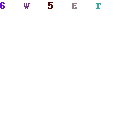Sierpinski Triangles

Sierpinski triangles and squares are relatively easy fractals to draw, and although the principle is the same I will handle the two separately.

For Sierpinski triangles, you take your initial triangle (an equilateral triangle works nicely) and then finding the midpoints of each side and connecting them, break up the triangle into four new triangles. Then for each of the three triangles on the outside, repeat this process until you reach the desired recursion depth. The middle triangle is left alone, and remains unfilled. When you reach the recursion depth you require, you can then colour the triangle the desired colour.Sierpinski Squares

Sierpinksi squares work in a similar way, except, instead of using the midpoint, you divide each edge into three pieces, and then split up the initial square into nine new squares. Repeat this process for the eight outer squares, leaving the middle square, as in for the triangles, alone. Once you reach the desired recursion depth, you then colour the square the desired colour.

So, then, the code to generate this is as follows. There is nothing tricky at all about it. In both cases, if the recursion depth is not yet met, the points are calculated, and the function is called recursively for each new shape, and if you have recursed far enough, then draw the shape based on the desired colour and coordinates. The coordinates of the initial shapes are passed as doubles to the functions, such as x1, y1 etc.

```        public void GenerateSquare(Graphics g, int iIterations, double x1, double y1, double x2, double y2, double x3, double y3, double x4, double y4, Color oColor)
{
if (iIterations > 1)
{
double midx1a = x1 + ((x2 - x1) / 3);
double midy1a = y1;
double midx1b = x1 + ((x2 - x1) / 3 * 2);
double midy1b = y1;
double midx2a = x2;
double midy2a = y2 + ((y3 - y2) / 3);
double midx2b = x2;
double midy2b = y2 + ((y3 - y2) / 3 * 2);
double midx3a = x1 + ((x3 - x4) / 3);
double midy3a = y3;
double midx3b = x1 + ((x3 - x4) / 3 * 2);
double midy3b = y3;
double midx4a = x1;
double midy4a = y1 + ((y4 - y1) / 3);
double midx4b = x1;
double midy4b = y1 + ((y4 - y1) / 3 * 2);
double midx5a = x1 + ((x2 - x1) / 3);
double midy5a = y1 + ((y4 - y1) / 3);
double midx5b = x1 + ((x2 - x1) / 3 * 2);
double midy5b = y1 + ((y4 - y1) / 3);
double midx5d = x1 + ((x2 - x1) / 3);
double midy5d = y1 + ((y4 - y1) / 3 * 2);
double midx5c = x1 + ((x2 - x1) / 3 * 2);
double midy5c = y1 + ((y4 - y1) / 3 * 2);

GenerateSquare(g, iIterations - 1, x1, y1, midx1a, midy1a, midx5a, midy5a, midx4a, midy4a, oColor);
GenerateSquare(g, iIterations - 1, midx1a, midy1a, midx1b, midy1b, midx5b, midy5b, midx5a, midy5a, oColor);
GenerateSquare(g, iIterations - 1, midx1b, midy1b, x2, y2, midx2a, midy2a, midx5b, midy5b, oColor);
GenerateSquare(g, iIterations - 1, midx5b, midy5b, midx2a, midy2a, midx2b, midy2b, midx5c, midy5c, oColor);
GenerateSquare(g, iIterations - 1, midx5c, midy5c, midx2b, midy2b, x3, y3, midx3b, midy3b, oColor);
GenerateSquare(g, iIterations - 1, midx5d, midy5d, midx5c, midy5c, midx3b, midy3b, midx3a, midy3a, oColor);
GenerateSquare(g, iIterations - 1, midx4b, midy4b, midx5d, midy5d, midx3a, midy3a, x4, y4, oColor);
GenerateSquare(g, iIterations - 1, midx4a, midy4a, midx5a, midy5a, midx5d, midy5d, midx4b, midy4b, oColor);
}
else
{
Pen o = new Pen(new SolidBrush(oColor));
PointF[] points = new PointF;
points = new PointF((float)x1, (float)y1);
points = new PointF((float)x2, (float)y2);
points = new PointF((float)x3, (float)y3);
points = new PointF((float)x4, (float)y4);

g.FillPolygon(new SolidBrush(oColor), points);
}

}

public void GenerateTriangle(Graphics g, int iIterations, double x1, double y1, double x2, double y2, double x3, double y3, Color oColor)
{

if (iIterations > 1)
{
double midx1 = (x1 + x2) / 2;
double midy1 = (y1 + y2) / 2;
double midx2 = (x2 + x3) / 2;
double midy2 = (y2 + y3) / 2;
double midx3 = (x3 + x1) / 2;
double midy3 = (y3 + y1) / 2;
GenerateTriangle(g, iIterations - 1, x1, y1, midx1, midy1, midx3, midy3, oColor);
GenerateTriangle(g, iIterations - 1, midx1, midy1, x2, y2, midx2, midy2, oColor);
GenerateTriangle(g, iIterations - 1, midx3, midy3, midx2, midy2, x3, y3, oColor);
}
else
{
PointF[] points = new PointF;
points = new PointF((float)x1, (float)y1);
points = new PointF((float)x2, (float)y2);
points = new PointF((float)x3, (float)y3);

g.FillPolygon(new SolidBrush(oColor), points);
}
}

```

You can get the full source code, which includes all the other fractals, here.